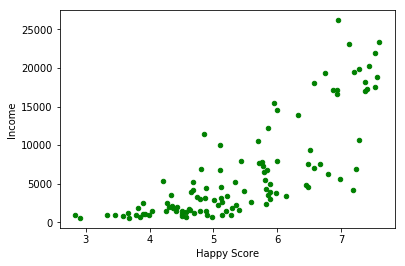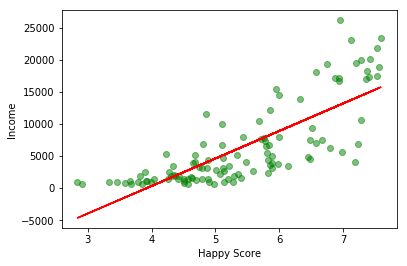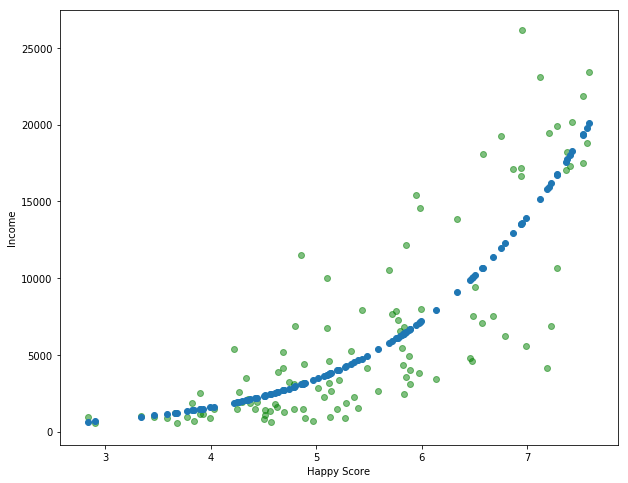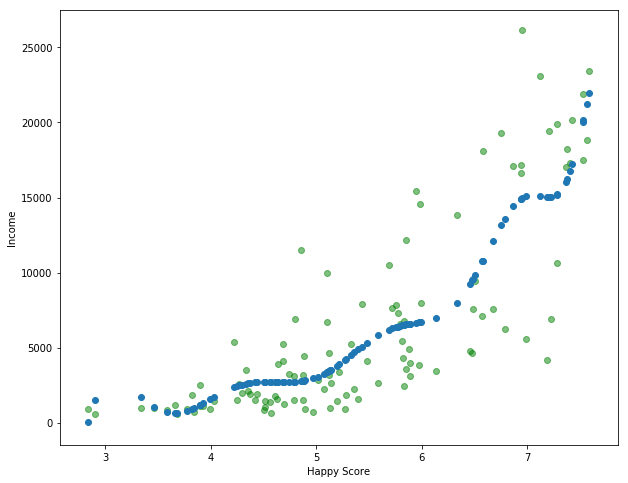In this short guide you can learn the basics of linear regression in Python. Linear regression is a model which represents the relation of variables - they can be independent or dependent. Linear regression can help to answer the question of dependency or numeric variables.

So let's explain it in a simple example. Suppose you are a data scientist which is asked:

Is there a relation between money and happiness?

To prove it you will need to do it in several steps:

• define the question (it's already done)
• find data
• analyse it
• summarize it

## Step 1: Find and prepare data for Linear Regression

Once the question is defined we can search for data. Then you may need to do some preprocessing of the data like:

• clean - bad records, incomplete data
• format issues

Suppose we have data like:

``````import pandas as pd
import numpy as np
import matplotlib as mpl
import matplotlib.pyplot as plt
from sklearn.linear_model import LinearRegression

# import and preview dataset
``````
Armenia 37.0 2096.76 4.350
Angola 26.0 1448.88 4.033
Argentina 60.0 7101.12 6.574
Austria 59.0 19457.04 7.200
Australia 65.0 19917.00 7.284

Data is taken from Kaggle and there's no need to clean or process it.

## Step 2: Scatterplot and Linear Regression

In this step we are going to plot simple Scatterplot in Python. We need two numeric variables like:

``````# basic plot scatter
plt.xlabel('Happy Score')
plt.ylabel('Income')
plt.scatter('happyScore', 'avg_income', data=df, s=20, color='green')
``````

result:Next step is to perform the linear regression. We are going to search for the relation between `happyScore` and `avg_income`. We are using class: `LinearRegression` from `sklearn.linear_model`

``````X = df['happyScore'].values.reshape(-1, 1)
Y = df['avg_income'].values.reshape(-1, 1)

linear_regressor = LinearRegression()
linear_regressor.fit(X, Y)
Y_pred = linear_regressor.predict(X)
``````

The result is array of predicted values like:

``````array([[ 1842.30538429],
[  481.79795859],
[11387.31647189],
[14073.99675104],
[14434.50975975],
[ 5541.85554504],
[ 3318.69199137],
``````

## Step 3: Plot Linear Regression in Python

Finally we are going to** plot the regression line on the scatterplot** from the previous step:

``````plt.xlabel('Happy Score')
plt.ylabel('Income')

plt.scatter(X, Y, color='green', alpha=0.5)
plt.plot(X, Y_pred, color='red')
plt.show()
``````

the result shows that there might be a relation between those two:## Step 4: Polynomial Linear Regression in Python

Finally let's check how to extend linear regression with polynomial features in Python. This approach is more precise in some cases:

``````from sklearn.preprocessing import PolynomialFeatures

# prepare Polynomial Features
pr = PolynomialFeatures(degree = 5)
X_poly = pr.fit_transform(X)
pr.fit(X_poly, Y)

# Polynomial Linear Regression
lin_reg = LinearRegression()
lin_reg.fit(X_poly, Y)

# plot
plt.scatter(X, Y, color='green', alpha=0.5)
plt.scatter(X, lin_reg.predict(pr.fit_transform(X)))
plt.show()
``````

The result:We are fitting the model with `degree = 5` which:

• degree equal to 1 will be the same as the linear regression
• higher degree will take more time to compute
• higher degree will match relation better

Example for degree 15: